# VLOOKUP Contains (Partial Match) – Excel & Google Sheets

This tutorial will demonstrate how to perform a partial match VLOOKUP in Excel.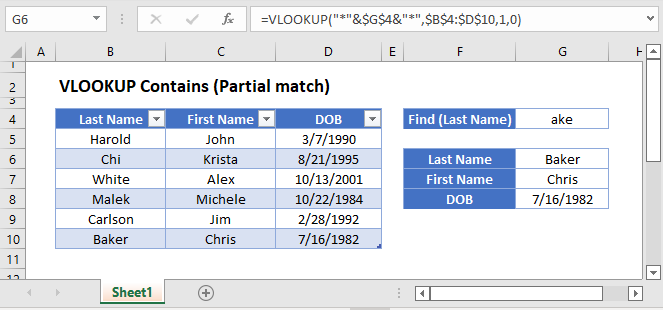## VLOOKUP Function

The VLOOKUP Function lookups a value in the leftmost column of a range and returns a corresponding value from another column.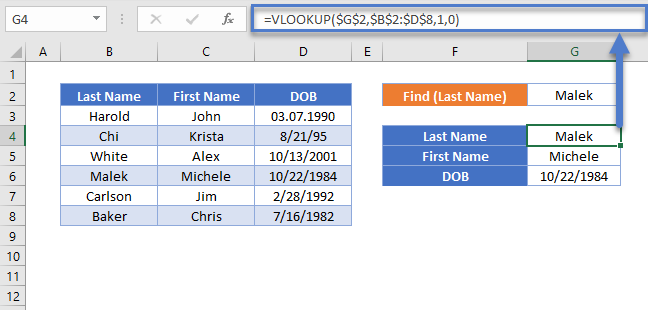## Partial Match VLOOKUP (Text Contains)

By using the asterisk “wildcard” (*), within a VLOOKUP, we can lookup values that contain (partial match) certain text, instead of values that match the lookup text exactly. Let’s walk through an example.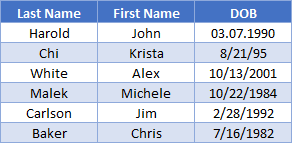Here we have a list of names and want to find a name that contains “ake”.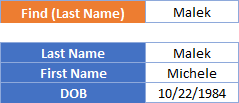To accomplish this, we combine VLOOKUP with a wildcard in a formula like so:

``=VLOOKUP("*"&<Partial Value>&"*",<lookup range>,<match column position>,0)``
``=VLOOKUP("*"&\$G\$2&"*",\$B\$2:\$D\$8,1,0)``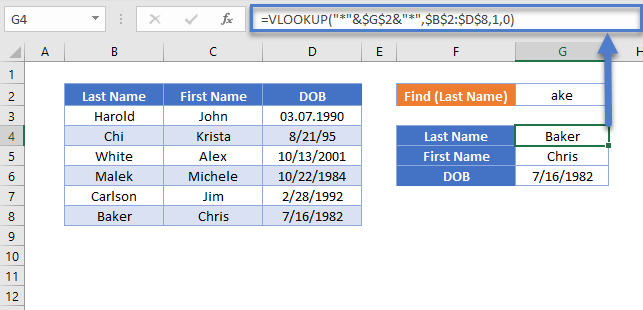The formula merges “ake” with the “*” wildcards, using the “&” symbol. Because the “*” wildcard is before and after the lookup text, the VLOOKUP Function will look for “ake” anywhere in the lookup range. Instead you could add “*” only before the text to lookup values that end in the lookup text. Or “*” after the lookup value for values that begin with the lookup text.

Limitation: wildcards only work with VLOOKUP function in exact mode (0 or FALSE for the [range lookup] parameter)

## VLOOKUP Contains (Partial Match) in Google Sheets

These formulas work exactly the same in Google Sheets as in Excel.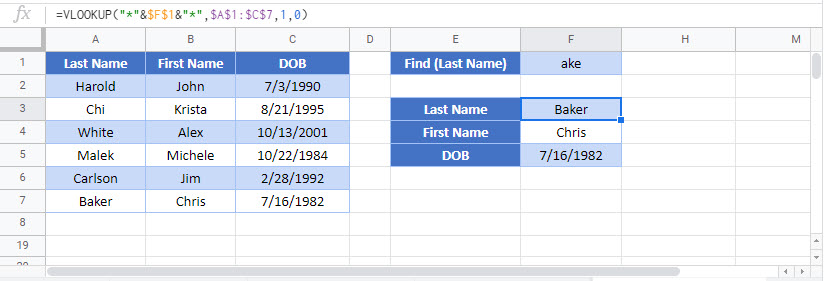### Excel Practice Worksheet

Practice Excel functions and formulas with our 100% free practice worksheets!